﻿ 基于LTE系统的改良接收信号功率测量方法文章快速检索 高级检索
 重庆邮电大学学报(自然科学版)2018, Vol. 30Issue (6): 746-751  DOI: 10.3979/j.issn.1673-825X.2018.06.0030

### 引用本文LI Zheng, OUYANG Weihua, JIANG Wenhao. Improvement of RSRP measurement method for LTE system[J]. Journal of Chongqing University of Posts and Telecommunications (Natural Science Edition), 2018, 30(6): 746-751. DOI: 10.3979/j.issn.1673-825X.2018.06.003.### 文章历史

1. 重庆邮电大学 移动通信工程研究中心，重庆 400065;
2. 重庆航天职业技术学院 计算机工程系，重庆 400021

Improvement of RSRP measurement method for LTE system
1. Mobile Communication Engineering Research Center, Chongqing University of Posts and Telecommunications, Chongqing 400065, P. R. China;
2. Computer Engineering Department, Chongqing Aerospace Polytechnic, Chongqing 400021, P. R. China
Foundation Items: The Science and Technology Research Program of Chongqing Municipal Education Commission(KJ1500443, KJ1704096, KJQN201800642, KJQN201803310)
Abstract: In long term evolution (LTE) system, the terminal is required to update the measurement information of same or neighboring cell to facilitate timely cell handover or reselection. Although the traditional received signal power measurement methods can reduce the influence of interference and noise on the signal at the receiving end, however, in LTE wireless environment, the reference signal receiving power(RSRP), which is often subject to time synchronization and frequency offset, is difficult to obtain precise measurement results. To solve this, an improved power measurement method of received signal based on both the structure of LTE system and measurement criterion is proposed. This algorithm requires low time and frequency synchronization, and the measurement performance of the received signal is improved slightly. The power measurement value of the received signal is calculated in frequency and time domain, respectively, and the larger of the two values is considered as the final received signal value. Simulation results show that the performance gain based on average measurement error of the proposed algorithm over traditional algorithm can achieve more than 1dB when the frequency offset is not more than 3 kHz, the time deviation is not more than 30 ns.
Keywords: long term evolution(LTE)    measurement    synchronous deviation    reference signal receiving power(RSRP)
0 引言

1 小区接收信号功率测量基础 1.1 LTE系统物理层结构

LTE是第三代合作伙伴计划(3rd generation partnership project，3GPP)组织制定的通用移动通信系统(universal mobile telecommunications system，UMTS)技术标准的长期演进。它引入了正交频分复用(orthogonal frequency division multiplexing，OFDM)和多输入多输出(multi-input & multi-output，MIMO)等关键技术，这显著增加了频谱效率和数据传输速率(峰值下载速度可高达299.6 Mbit/s，峰值上传速度可高达75.4 Mbit/s)

LTE的无线接口，即用户终端(user equipment，UE)和网络之间的接口，包括L1，L2和L3，如图 1所示。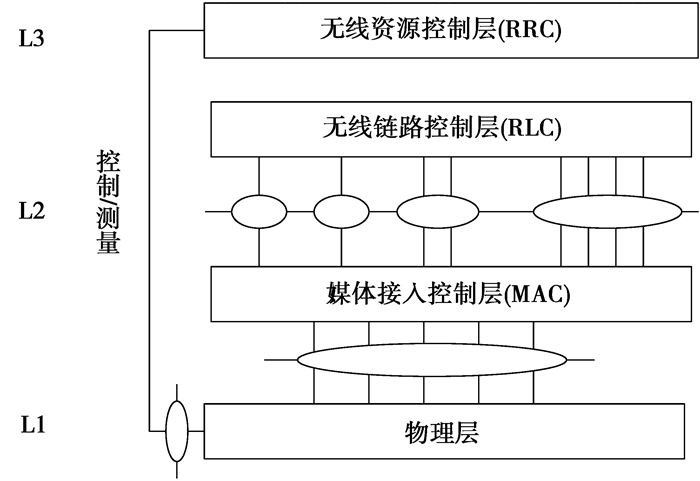图 1 LTE无线接口协议体系结构 Figure 1 LTE wireless interface protocol architecture

LTE系统中，最小的时域大小定义为，Ts=1/(15 000×2 048)s，各种域的时域大小均为Ts的倍数。

LTE系统的类型1帧结构，如图 2所示。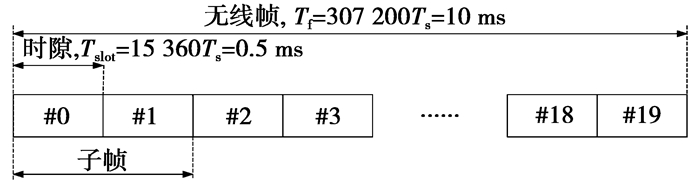图 2 类型1帧结构 Figure 2 Frame structure of type 1

LTE系统中，每一个无线帧的长度为10 ms，无线帧包括了若干子帧，其中常规子帧长度为1 ms，每个常规子帧有14个OFDM符号，数据调制到OFDM符号的频域上，频域间隔为15 kHz。在LTE系统的多种系统带宽中，随着带宽的增加，可承载的数据也会更多。随着采样率的不同，一个符号包含的采样点个数也不同。不失一般性，本文以20 MHz为例，则可调制1 200个数据，采样率为30.72 MHz，那么一个符号包括了2 048个采样点。用于终端信道估计和小区测量的小区参考信号(简称Cell RS)位于下行常规子帧的14个符号中的4个符号，间隔为90 kHz，在频域中的位置及数值由小区ID号、子帧号、Cell RS所处14个符号中的位置确定，总共有200个数据[4-7]

1.2 RSRP测量

2 LTE接收信号功率测量算法

2.1 频域接收信号功率测量

rec_r_freq(n)除以本地训练序列频域数据rec_b_freq(n)获得频域比值序列

 $freq\_ratio\left( n \right)=\frac{rec\_r\_freq\left( n \right)}{rec\_b\_freq\left( n \right)}$ (1)

freq_radio(n)按不同的间隔k进行滑动自相关，其中，k=1, 2, …, n-1，表示参与计算的样点是否连续或间隔，获得频域滑动相关值序列

 $\begin{matrix} freq\_xcoor\left( k \right)= \\ \frac{\sum\limits_{n=1}^{N-K}{freq\_radio\left( n+k \right)*\overline{freq\_radio\left( n \right)}}}{N-K} \\ \end{matrix}$ (2)

(2) 式中，*号表示卷积。

 $freq\_xcoor\_power\left( k \right)=\left| freq\_xcoor\left( k \right) \right|$ (3)

 $EF\_xcoor\_power=\frac{\sum\limits_{k=1}^{K}{freq\_xcoor\_power\left( k \right)}}{K}$ (4)

(4) 式中，k=1, 2, …, KK∈(0, N)。

2.2 时域接收信号功率测量

rec_r_time(n)除以本地训练序列时域数据rec_b_time(n)获得时域比值序列

 $time\_ratio\left( n \right)=\frac{rec\_r\_time\left( n \right)}{rec\_b\_time\left( n \right)}$ (5)

time_ratio(n)按不同的间隔k进行滑动自相关，其中，k=1, 2, …, n-1，表示参与计算的样点是否连续或间隔，获得时域滑动相关值序列

 $\begin{matrix} time\_xcoor\left( k \right) \\ \frac{\sum\limits_{n=1}^{N-K}{time\_radio\left( n+k \right)*\overline{time\_radio\left( n \right)}}}{N-K} \\ \end{matrix}$ (6)

 $time\_xcoor\_power\left( k \right)=\left| time\_xcoor\left( k \right) \right|$ (7)

 $ET\_xcoor\_power=\frac{\sum\limits_{k=1}^{K}{time\_xcoor\_power\left( k \right)}}{K}$ (8)

(8) 式中，k=1, 2, …, KK∈(0, N)。

2.3 时域频域协同接收信号测量

 \begin{align} &Cell\_power= \\ &\text{max}\left( EF\_xcoor\_power, ET\_xcoor\_power \right) \\ \end{align} (9)

 $r\left( t \right)=\text{sqrt}\left( P \right)\cdot b\left( t \right)\cdot {{\text{e}}^{\text{j}\mathit{wt}}}+n\left( t \right)$ (10)

(10) 式中：r(t)表示接收信号；b(t)表示本地信号；P表示待测量功率；ejwt表示频偏影响；n(t)表示噪声。

 $r\left( t \right)/b\left( t \right)=\text{sqrt}\left( P \right)\cdot {{\text{e}}^{\text{j}\mathit{wt}}}+n\left( t \right)/b\left( t \right)$ (11)

 \begin{align} &P\_est=\frac{{{\left| \sum\limits_{t=1}^{T}{r\left( t \right)/b\left( t \right)} \right|}^{2}}}{T}= \\ &\ \ \ \ \ \ \ \ \ \ \ \ \frac{{{\left| \text{sqrt}\left( p \right)\sum\limits_{t=1}^{T}{{{\text{e}}^{\text{j}\mathit{wt}}}+{n}'\left( t \right)} \right|}^{2}}}{T} \\ \end{align} (12)

(12) 式中，n′(t)为可由序列长度增加而抵消的含噪声的项。而ejwt为频偏造成的影响，由于序列各索引值t不同，相应叠加无法抵消，使得计算功率值变低，从而影响真实结果。

 \begin{align} &P\_est= \\ &\frac{\left| \sum\limits_{t=1}^{T-I}{r\left( t+I \right)/b\left( t+I \right)*\overline{\left( r\left( t \right)/b\left( t \right) \right)}} \right|}{T} =\\ &\frac{\left| p\sum\limits_{t=1}^{T}{{{\text{e}}^{\text{j}\mathit{wI}}}+{n}''\left( t \right)} \right|}{T} \\ \end{align} (13)

(13) 式中，n″(t)为可由序列长度增加而抵消的含噪声的项。由ejwI频偏造成的影响，是一固定I值，I是采样点之间的固定频率偏差，其固定值取决于滑动相关间隔k，不受序列索引值影响，因此，相应叠加也不会影响信号功率的测量。

3 仿真与结果分析 3.1 仿真设置表 1 仿真参数设置 Table 1 Simulation parameters setting

3.2 仿真结果分析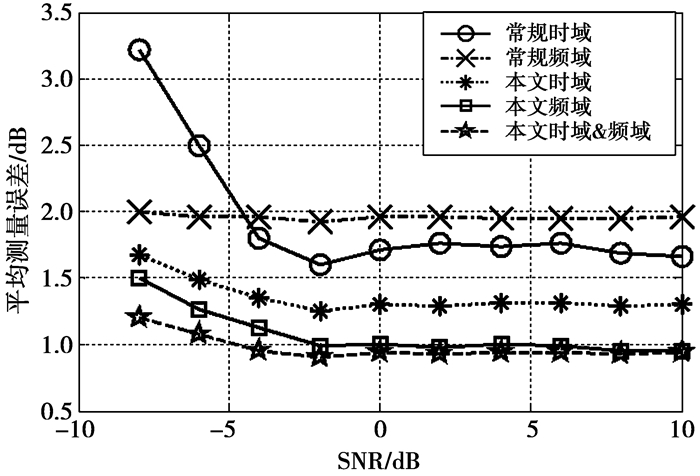图 3 测试环境1仿真结果 Figure 3 Results of simulation test environment 1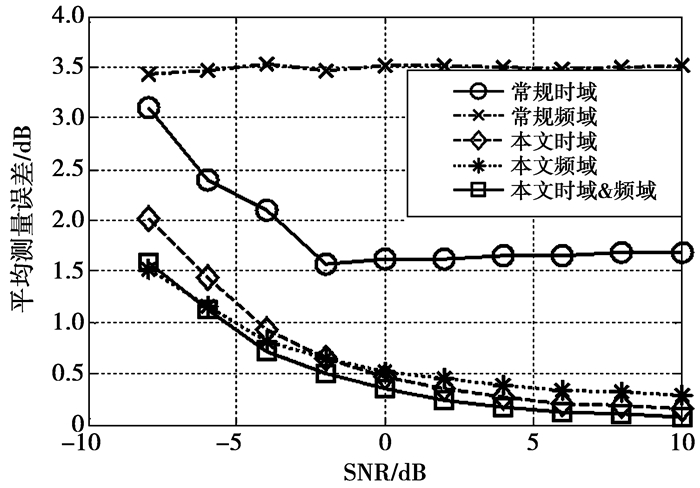图 4 测试环境2仿真结果 Figure 4 Results of simulation test environment 2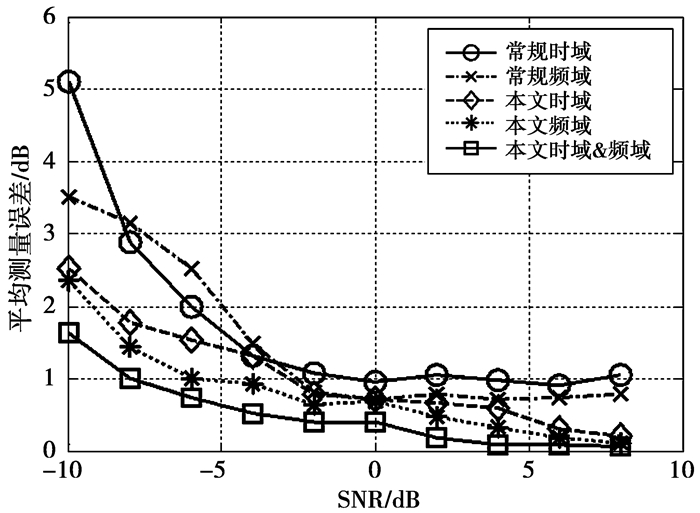图 5 测试环境3仿真结果 Figure 5 Results of simulation test environment 3

3.3 计算复杂度分析表 2 算法复杂度对比 Table 2 Comparative of algorithm complexity

4 结束语

  董晶. LTE系统中下行信号质量RSRP测量研究[J]. 科技信息, 2012(9): 79-80. DONG Jing. Research on Downlink Signal Quality RSRP Measurement in LTE System[J]. Science & Technology Information, 2012(9): 79-80.  SRIHARSHA M R, DAMA S, KUCHI K. A complete cell search and synchronization in LTE[J]. Eurasip Journal on Wireless Communications & Networking, 2017(1): 101-114.  3GPP.TS 36.508, Common test environments for User Equipment (UE)[EB/OL].(2017-03-23)[2017-10-12].http://www.3gpp.org/ftp/specs/archive/36_series/36.508/.  COPET P B, MARCHETTO G, SISTO R, et al. Formal Verification of LTE-UMTS and LTE-LTE Handover Procedures[J]. Computer Standards & Interfaces, 2016, 50(C): 92-106.  李阳, 李小文, 韩娜娜. TD-LTE系统RRC层测量过程分析与实现[J]. 广东通信技术, 2014, 34(6): 37-41. LI Yang, LI Xiaowen, HAN Nana. Analysis and Implementation of RRC Layer Measurement Process in TD-LTE System[J]. Guangdong Communication Technology, 2014, 34(6): 37-41. DOI:10.3969/j.issn.1006-6403.2014.06.008  陈发堂, 郑晓献. TD-LTE系统UE端EVM测量与DSP实现[J]. 光通信研究, 2011(6): 63-66. CHEN Fatang, ZHENG Xiaoxian. EVM measurement and DSP realization for UE of TD-LTE system[J]. Study on Optical Communications, 2011(6): 63-66. DOI:10.3969/j.issn.1005-8788.2011.06.019  杨丰瑞, 周侃. 基于LTE小区重选的RSRP测量研究[J]. 电子测试, 2010(8): 45-50. YANG Fengrui, ZHANG Kan. Research of RSRP for LTE reselection[J]. Electronic Test, 2010(8): 45-50. DOI:10.3969/j.issn.1000-8519.2010.08.010  MAHFOUDI M, BEKKALI M E, NAJD A, et al. A New Downlink Scheduling Algorithm Proposed for Real Time Traffic in LTE System[J]. International Journal of Electronics & Telecommuni- cations, 2015, 61(4): 409-414.  KRUAJIRAYU H, TANGSOMBOON A, LEELANUPAB T.CoZpace: A proposal for collaborative web search for sharing search records and interactions[C]//Student Project Conference.New York: IEEE Press, 2014: 165-168. http://ieeexplore.ieee.org/xpls/abs_all.jsp?arnumber=6923242  胡亚希, 梁双春, 方媛. 基于LTE测量报告数据的小区覆盖分析[J]. 电信工程技术与标准化, 2013, 26(1): 33-37. HU Yaxi, LIANG Shuangchun, FANG Yuan. Cell coverage analysis based on LTE measurement report data[J]. Telecom Engineering Technics and Standardization, 2013, 26(1): 33-37. DOI:10.3969/j.issn.1008-5599.2013.01.010  王嘎, 张志超, 周薛雪. 一种基于线性正则变换域功率谱的信号检测方法[J]. 四川大学学报:自然科学版, 2016, 53(4): 723-730. WANG Ga, ZHANG Zhichao, ZHOU Xuexue. Signal Detection based on the Linear Canonical Transform domain Power Spectrum[J]. Journal of Sichuan University:Natural Science Edition, 2016, 53(4): 723-730.  王丹, 石伟萍, 李小文. LTE系统小区搜索中SSS时频同步算法的研究[J]. 重庆邮电大学学报:自然科学版, 2013, 25(6): 720-725. WANG Dan, SHI Weiping, LI Xiaowen. Time and frequency synchronization algorithm based on SSS of cell search in TD-LTE system[J]. Journal of Chongqing University of Post and Telecommunications:Natural Science Edition, 2013, 25(6): 720-725.  王玄, 彭端, 张雅媛. 基于LTE-Advanced上行链路的多级迭代干扰消除检测算法[J]. 重庆邮电大学学报:自然科学版, 2015, 27(6): 758-761. WANG Xuan, PENG Duan, ZHANG Yayuan. Multi-stage iteration interference cancellation algorithm based on LTE-Advanced uplink[J]. Journal of Chongqing University of Post and Telecommunications:Natural Science Edition, 2015, 27(6): 758-761.